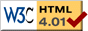# Single Spring

previous next
• Sim
• Graph
• Time Graph
• Multi Graph

This simulation shows a single mass on a spring, which is connected to a wall. This is an example of a simple linear oscillator.

You can change mass, spring stiffness, and friction (damping). You can drag the mass with your mouse to change the starting position.

The math behind the simulation is shown below. Also available are: open source code, documentation and a simple-compiled version which is more customizable.

## Puzzles

Try using the graph and changing parameters like mass or spring stiffness to answer these questions:

• What is the relationship between acceleration and position?
• How do mass or spring stiffness affect the relationship between acceleration and position?
• How do mass or spring stiffness affect the period or frequency of the oscillation?

## Physics

We define the following variables and constants

• x = position of the block
• v = x' = velocity of the block
• m = mass of the block
• R = rest length of the spring
• k = spring stiffness
• b = damping constant (friction)

A spring generates a force proportional to how far it is stretched (and acting in the opposite direction to the stretch)

Fspring = −k × stretch

If we adjust the coordinate system so that x = 0 corresponds to the spring being unstretched, then the stretch of the spring is simply equal to x . The spring force becomes

Fspring = − k x

In addition, there is a damping (friction) force that resists the motion. It is proportional to the velocity. So we add Fdamping = −b v to get the total force

F = Fspring + Fdamping = − k xb v

Combining this with Newton's law of motion F = m a , and the definition of acceleration as the second derivative of position a = x'' we have the differential equation:

m x'' = −k xb v

or equivalently:

 x'' = − k⁄m x − b⁄m x' (1)

Equation (1) is the equation of motion for the spring, defining exactly what happens over time.

You can actually see equation (1) visually if you turn on the graph in the simulation. Suppose there is no damping (set damping to zero). Then if you set the graph on the simulation to plot acceleration against position, you will get a straight line, with slope = −k/m . So if you increase the stiffness of the spring, the line becomes steeper. If you increase the mass, the line becomes less steep.

## Numerical Solution

To solve this equation numerically (ie. by computer) we use the Runge-Kutta method. To do so we need to convert the second order differential equation (1) into a set of first order differential equations. Note that we can write the acceleration as the first derivative of velocity: x'' = v' . Therefore we can express equation (1) as a system of two first order differential equations:

x' = v

v' = − km xbm v

This is the form that we need in order to use the Runge-Kutta method for numerically solving the differential equation.

To begin the simulation, we initialize the two variables x,v for their value at time t=0 . Then we use the Runge-Kutta algorithm to calculate the values of x,v after a short time interval, and this continues indefinitely.

## Analytic Solution

An analytic solution uses mathematics to find the solution instead of the brute force of the computer. The advantage is that we can get some more insight from the analytic solution, instead of having to analyze zillions of numbers that come from a numerical solution.

With no damping ( b = 0 ) and the block initially not moving, the analytic solution is given by

$$x(t) = x_0 \cos(\sqrt{k/m} \; t)$$

where x0 = initial position of the block and t = time. The period of the oscillation is the time it takes to repeat. From the solution we see that the oscillation repeats when $$\sqrt{k/m} \; t = 2 \pi$$, and so the period is

$$t = 2 \pi \sqrt{m/k}$$

The frequency is the inverse of the period:

$$frequency = \frac{1}{2 \pi} \sqrt{k/m}$$

So we predict that

• increasing mass by 4 times doubles the period and halves the frequency;
• increasing spring stiffness by 4 times halves the period and doubles the frequency;

You can check these predictions by modifying the parameters on the simulation (you'll need a stopwatch to time the frequency).

You can view a page with the derivation of the analytic solution.

What is the relationship between acceleration and position?

Answer: It is a linear relationship as given by the equation

x'' = − km x

where x = position, x'' = acceleration, m = mass, and k = spring stiffness.

How do mass or spring stiffness affect the relationship between acceleration and position?

x'' = − km x

we know that there is a simple linear relationship between acceleration and position. The mass and spring stiffness affect the slope of this line.

• Increasing mass makes the line less steep.
• Increasing spring stiffness makes the line steeper.

How do mass or spring stiffness affect the period or frequency of the oscillation?

$$x(t) = x_0 \cos(\sqrt{k/m} \; t)$$

and the frequency is given by

$$frequency = \frac{1}{2 \pi} \sqrt{k/m}$$

So we predict that

• increasing mass by 4 times doubles the period and halves the frequency;
• increasing spring stiffness by 4 times halves the period and doubles the frequency;

This web page was first published April 2001.

previous next